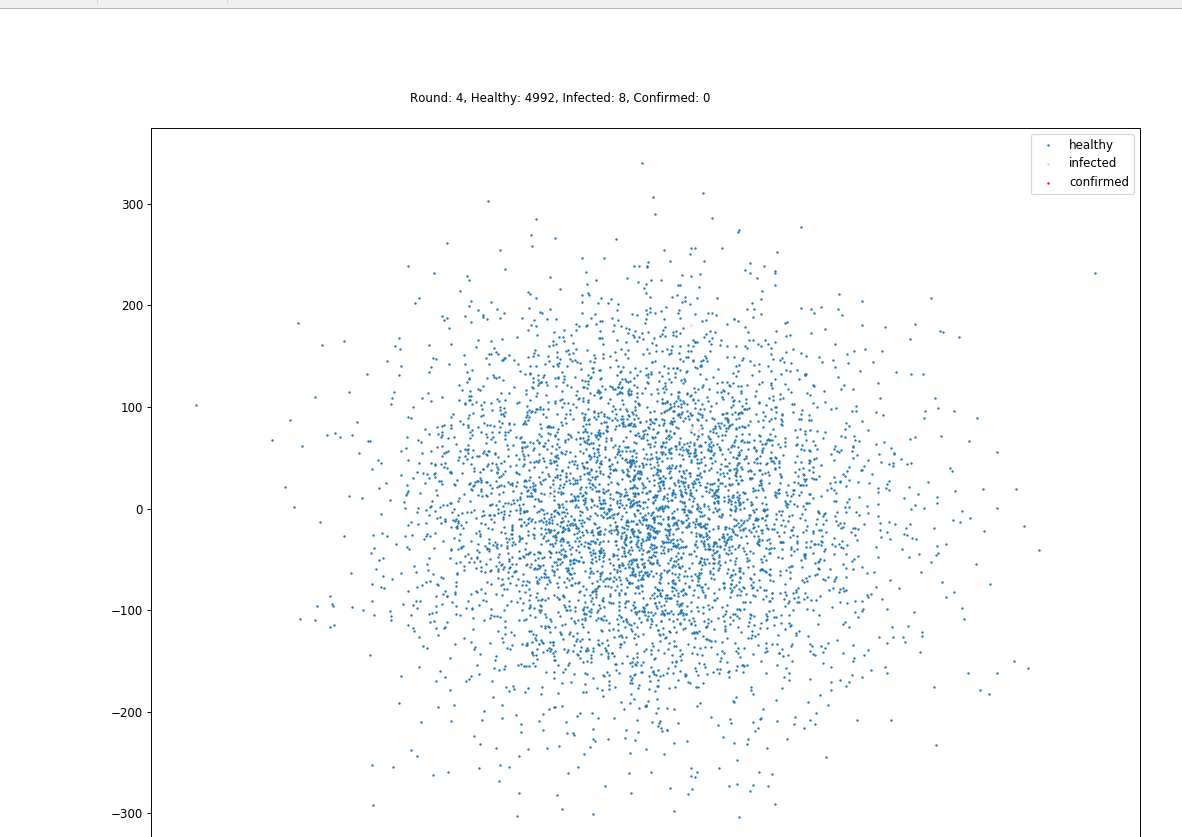# 尝试用 Python 写了个病毒传播模拟程序

2019/04/10 10:10

## Java 版程序浅析

public class Person extends Point {
private int state = State.NORMAL;
}


• 移动
• 状态变化
• 影响他人

## Python 版思路

import numpy as np
import matplotlib.pyplot as plt


## 如何模拟人群

class People(object):
def __init__(self, count=1000, first_infected_count=3):
self.count = count
self.first_infected_count = first_infected_count
self.init()


    def init(self):
self._people = np.random.normal(0, 100, (self.count, 2))
self.reset()


    def reset(self):
self._round = 0
self._status = np.array( * self.count)
self._timer = np.array( * self.count)
self.random_people_state(self.first_infected_count, 1)


    def random_people_state(self, num, state=1):
"""随机挑选人设置状态
"""
assert self.count > num
# TODO：极端情况下会出现无限循环
n = 0
while n < num:
i = np.random.randint(0, self.count)
if self._status[i] == state:
continue
else:
self.set_state(i, state)
n += 1

def set_state(self, i, state):
self._status[i] = state
# 记录状态改变的时间
self._timer[i] = self._round


    @property
def healthy(self):
return self._people[self._status == 0]

@property
def infected(self):
return self._people[self._status == 1]


    def update(self):
"""每一次迭代更新"""
self.change_state()
self.affect()
self.move()
self._round += 1
self.report()


### 如何改变状态

    def change_state(self):
dt = self._round - self._timer
# 必须先更新时钟再更新状态
d = np.random.randint(3, 5)
self._timer[(self._status == 1) & ((dt == d) | (dt > 14))] = self._round
self._status[(self._status == 1) & ((dt == d) | (dt > 14))] += 1


### 如何影响他人

    def infect_possible(self, x=0., safe_distance=3.0):
"""按概率感染接近的健康人
x 的取值参考正态分布概率表，x=0 时感染概率是 50%
"""
for inf in self.infected:
dm = (self._people - inf) ** 2
d = dm.sum(axis=1) ** 0.5
sorted_index = d.argsort()
for i in sorted_index:
if d[i] >= safe_distance:
break  # 超出范围，不用管了
if self._status[i] > 0:
continue
if np.random.normal() > x:
continue
self._status[i] = 1
# 记录状态改变的时间
self._timer[i] = self._round


### 如何移动

_people 是一个坐标矩阵，只要生成移动距离矩阵 dt，然后它相加即可。我们可以设置一个可移动的范围 width，把移动距离控制在一定范围内。

    def move(self, width=1, x=.0):
movement = self.random_movement(width=width)
# 限定特定状态的人员移动
switch = self.random_switch(x=x)
movement[switch == 0] = 0
self._people = self._people + movement


    def random_switch(self, x=0.):
"""随机生成开关，0 - 关，1 - 开

x 大致取值范围 -1.99 - 1.99；
对应正态分布的概率， 取值 0 的时候对应概率是 50%
:param x: 控制开关比例
:return:
"""
normal = np.random.normal(0, 1, self.count)
switch = np.where(normal < x, 1, 0)
return switch


### 输出结果

    def report(self):
plt.cla()
# plt.grid(False)
p1 = plt.scatter(self.healthy[:, 0], self.healthy[:, 1], s=1)
p2 = plt.scatter(self.infected[:, 0], self.infected[:, 1], s=1, c='pink')
p3 = plt.scatter(self.confirmed[:, 0], self.confirmed[:, 1], s=1, c='red')

plt.legend([p1, p2, p3], ['healthy', 'infected', 'confirmed'], loc='upper right', scatterpoints=1)
t = "Round: %s, Healthy: %s, Infected: %s, Confirmed: %s" % \
(self._round, len(self.healthy), len(self.infected), len(self.confirmed))
plt.text(-200, 400, t, ha='left', wrap=True)


## 实际效果

if __name__ == '__main__':
np.random.seed(0)
plt.figure(figsize=(16, 16), dpi=100)
plt.ion()
p = People(5000, 3)
for i in range(100):
p.update()
p.report()
plt.pause(.1)
plt.pause(3)## 后记

0
0 收藏

### 作者的其它热门文章0 评论
0 收藏
0